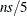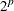### k-d Trees and Blending

PROC LOESS uses a k-d tree to divide the box (also called the initial cell or bucket) enclosing all the predictor data points into rectangular cells. The vertices of these cells are the points at which local least squares fitting is done.

Starting from the initial cell, the direction of the longest cell edge is selected as the split direction. The median of this coordinate of the data in the cell is the split value. The data in the starting cell are partitioned into two child cells. The left child consists of all data from the parent cell whose coordinate in the split direction is less than the split value. This procedure is repeated for each child cell that has more than a prespecified number of points, called the bucket size of the k-d tree.

You can specify the bucket size with the BUCKET= option in the MODEL statement. If you do not specify the BUCKET= option, the default value used is the largest integer less than or equal to, where n is the number of observations and s is the value of the smoothing parameter. Note that if fitting is being done for a range of smoothing parameter values, the bucket size can change for each value.

The set of vertices of all the cells of the k-d tree are the points at which PROC LOESS performs its local fitting. The fitted value at an original data point (or at any other point within the original data cell) is obtained by blending the fitted values at the vertices of the k-d tree cell that contains that data point.

The univariate blending methods available in PROC LOESS are linear and cubic polynomial interpolation, with linear interpolation being the default. You can request cubic interpolation by specifying the INTERP=CUBIC option in the MODEL statement. In this case, PROC LOESS uses the unique cubic polynomial whose values and first derivatives match those of the fitted local polynomials evaluated at the two endpoints of the k-d tree cell edge.

In the multivariate case, such univariate interpolating polynomials are computed on each edge of the k-d tree cells and are combined using blending functions (Gordon, 1971). In the case of two regressors, if you specify INTERP=CUBIC in the MODEL statement, PROC LOESS uses Hermite cubic polynomials as blending functions. If you do not specify INTERP=CUBIC, or if you specify a model with more than two regressors, then PROC LOESS uses linear polynomials as blending functions. In these cases, the blending method reduces to tensor product interpolation from thevertices of each k-d tree cell, where p is the number of regressors.

While the details of the k-d tree and the fitted values at the vertices of the k-d tree are implementation details that seldom need to be examined, PROC LOESS does provide options for their display. Each k-d tree subdivision of the data used by PROC LOESS is placed in the kdTree table. The predicted values at the vertices of each k-d tree are placed in the PredAtVertices table. You can request these tables by using the DETAILS option in the MODEL statement.